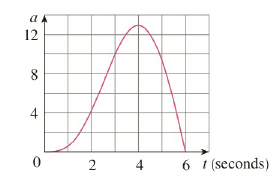Chapter 4.4, Problem 65E

Chapter
Section
Textbook Problem

# The graph of the acceleration a ( t ) of a car measured in ft/s 2 is shown. Use the Midpoint Rule to estimate the increase in the velocity of the car during the six-second time interval.To determine

To estimate:

The increase in the velocity of the car during the six-second time interval.

Explanation

1) Concept:

Use the Net Change theorem and the Midpoint Rule.

2) Theorem and Rule:

Net Change theorem: The integral of a rate of change is the net change.

abF'(x)dx=Fb-F(a)

The Midpoint Rule:

abfxdxi=1nfxi- x=x fx1-++fxn-

where x=b-an and xi-=12xi-1+xi midpoint of xi-1, xi.

2) Calculation:

Acceleration is the rate of change of velocity v with respect to time t.

at=v'(t)

Here t0,6

By Net Change theorem,

06at dt06v'tdt=v6-v(0)

is the change in velocity of the car during the given time interval.

Approximate the value of the integral using the Midpoint Rule with three subintervals: that is with n=3, a=0 and b=6 So width of the interval is

t=b-an

Substitute values

### Still sussing out bartleby?

Check out a sample textbook solution.

See a sample solution

#### The Solution to Your Study Problems

Bartleby provides explanations to thousands of textbook problems written by our experts, many with advanced degrees!

Get Started

#### Rationalize the denominator and simplify: 1052

Precalculus: Mathematics for Calculus (Standalone Book)

#### 165/8161/2167/8

Applied Calculus for the Managerial, Life, and Social Sciences: A Brief Approach

#### The interval of convergence of is: [−1, 1] [−1, 1) (−1, 1] (−1, 1)

Study Guide for Stewart's Single Variable Calculus: Early Transcendentals, 8th